Скачать презентацию International Corporate Finance ICF Jim Cook-Hauptman Associates Inc

0579b85fd60528d94f1c8f6f0281b5a8.ppt

• Количество слайдов: 25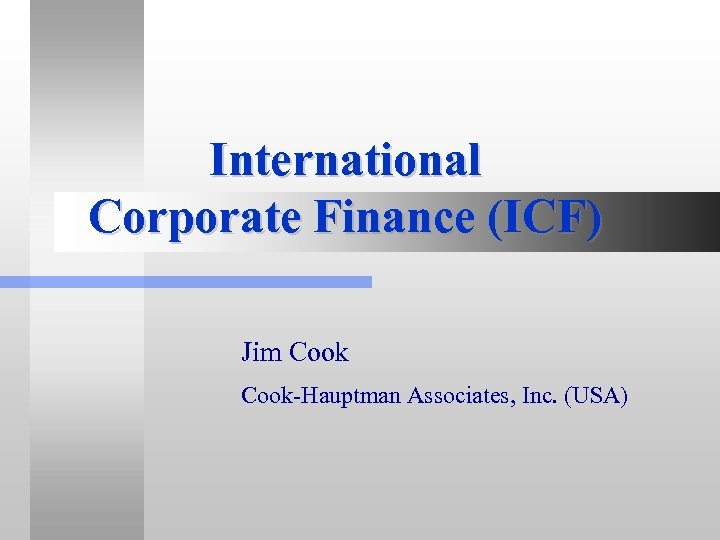International Corporate Finance (ICF) Jim Cook-Hauptman Associates, Inc. (USA)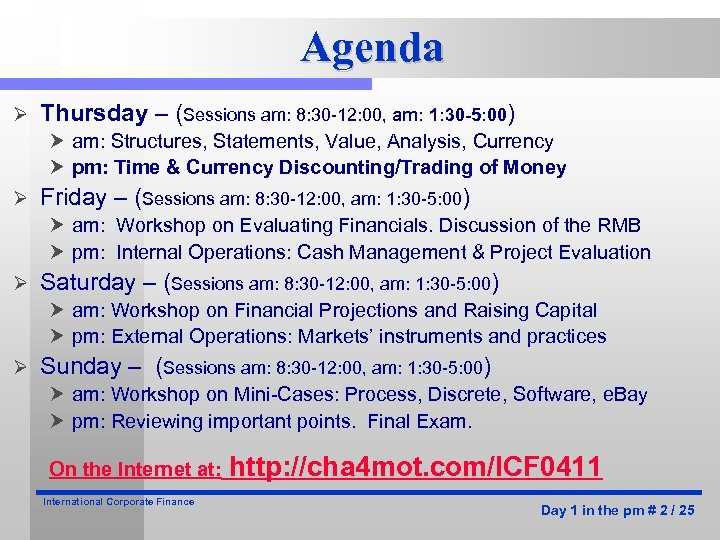Agenda Ø Thursday – (Sessions am: 8: 30 -12: 00, am: 1: 30 -5: 00) am: Structures, Statements, Value, Analysis, Currency pm: Time & Currency Discounting/Trading of Money Ø Friday – (Sessions am: 8: 30 -12: 00, am: 1: 30 -5: 00) am: Workshop on Evaluating Financials. Discussion of the RMB pm: Internal Operations: Cash Management & Project Evaluation Ø Saturday – (Sessions am: 8: 30 -12: 00, am: 1: 30 -5: 00) am: Workshop on Financial Projections and Raising Capital pm: External Operations: Markets’ instruments and practices Ø Sunday – (Sessions am: 8: 30 -12: 00, am: 1: 30 -5: 00) am: Workshop on Mini-Cases: Process, Discrete, Software, e. Bay pm: Reviewing important points. Final Exam. On the Internet at: International Corporate Finance http: //cha 4 mot. com/ICF 0411 Day 1 in the pm # 2 / 25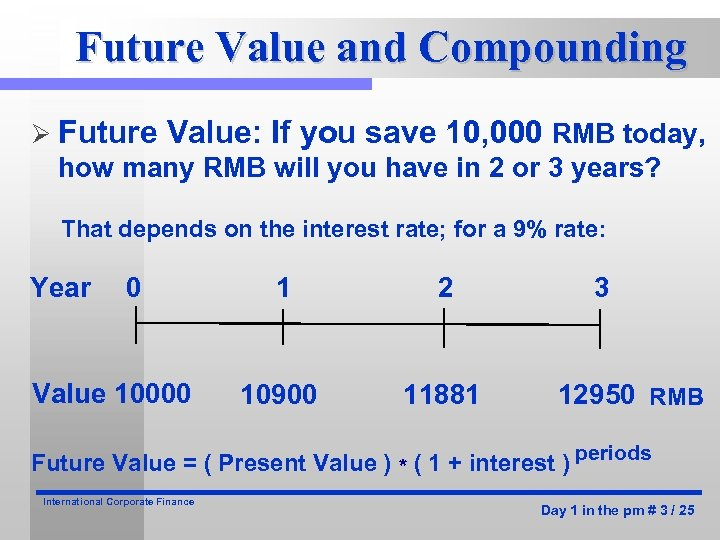Future Value and Compounding Ø Future Value: If you save 10, 000 RMB today, how many RMB will you have in 2 or 3 years? That depends on the interest rate; for a 9% rate: Year 0 Value 10000 1 2 10900 11881 3 12950 RMB Future Value = ( Present Value ) * ( 1 + interest ) periods International Corporate Finance Day 1 in the pm # 3 / 25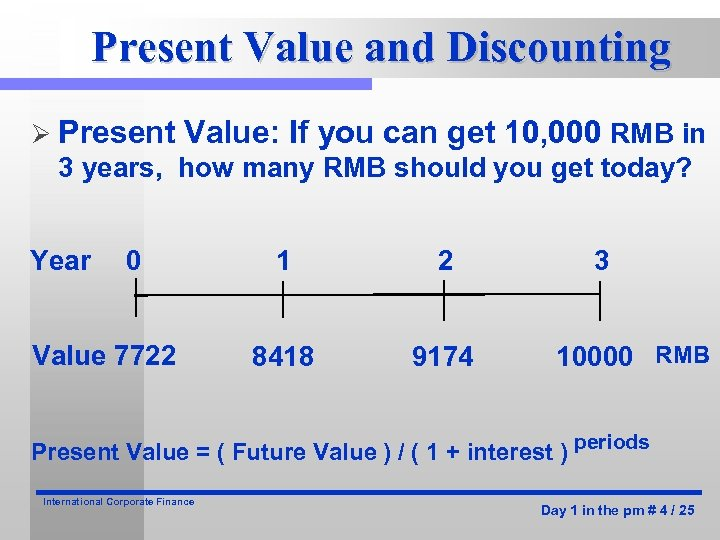Present Value and Discounting Ø Present Value: If you can get 10, 000 RMB in 3 years, how many RMB should you get today? Year 0 Value 7722 1 2 8418 9174 3 10000 RMB Present Value = ( Future Value ) / ( 1 + interest ) periods International Corporate Finance Day 1 in the pm # 4 / 25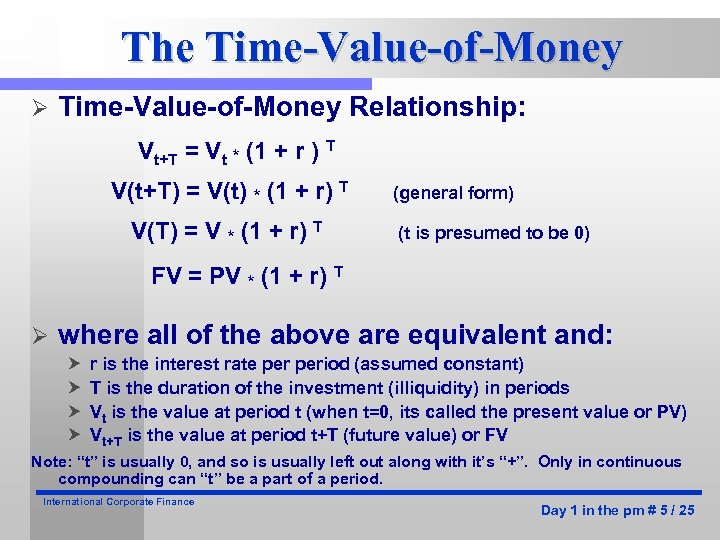The Time-Value-of-Money Ø Time-Value-of-Money Relationship: Vt+T = Vt * (1 + r ) T V(t+T) = V(t) * (1 + r) T V(T) = V * (1 + r) T (general form) (t is presumed to be 0) FV = PV * (1 + r) T Ø where all of the above are equivalent and: r is the interest rate period (assumed constant) T is the duration of the investment (illiquidity) in periods Vt is the value at period t (when t=0, its called the present value or PV) Vt+T is the value at period t+T (future value) or FV Note: “t” is usually 0, and so is usually left out along with it’s “+”. Only in continuous compounding can “t” be a part of a period. International Corporate Finance Day 1 in the pm # 5 / 25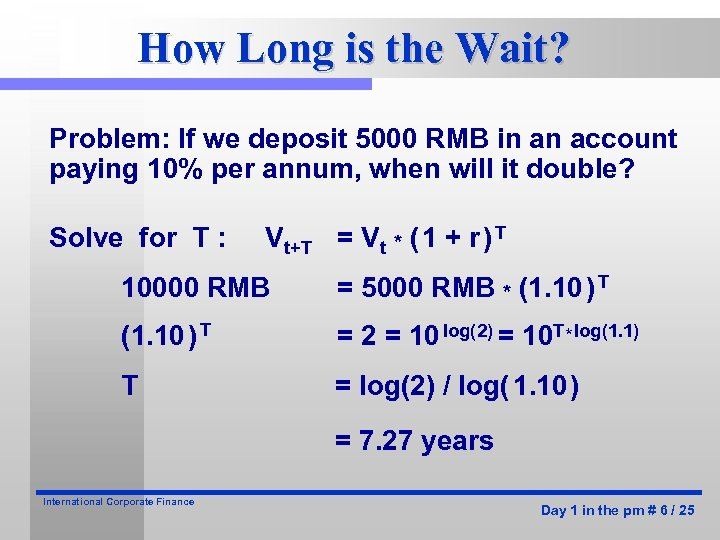How Long is the Wait? Problem: If we deposit 5000 RMB in an account paying 10% per annum, when will it double? Solve for T : Vt+T = Vt * ( 1 + r ) T 10000 RMB = 5000 RMB * (1. 10 ) T = 2 = 10 log(2) = 10 T * log(1. 1) T = log(2) / log( 1. 10 ) = 7. 27 years International Corporate Finance Day 1 in the pm # 6 / 25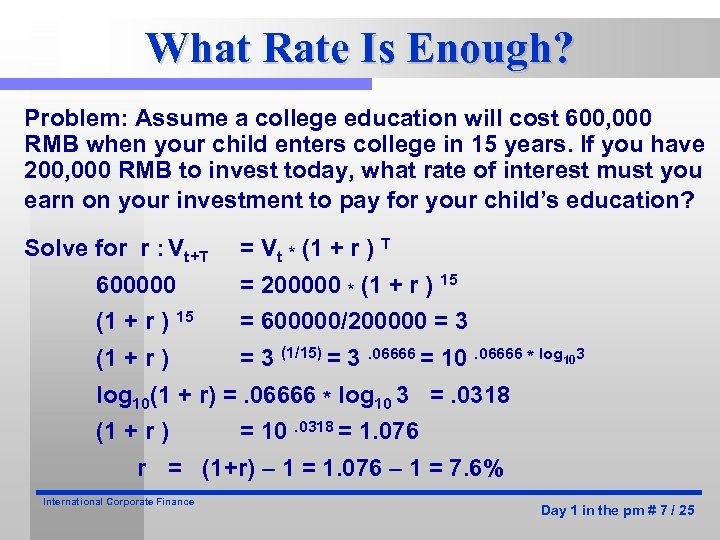What Rate Is Enough? Problem: Assume a college education will cost 600, 000 RMB when your child enters college in 15 years. If you have 200, 000 RMB to invest today, what rate of interest must you earn on your investment to pay for your child’s education? Solve for r : Vt+T = Vt * (1 + r ) T 600000 = 200000 * (1 + r ) 15 = 600000/200000 = 3 (1 + r ) = 3 (1/15) = 3. 06666 = 10. 06666 * log 103 log 10(1 + r) =. 06666 * log 10 3 =. 0318 (1 + r ) = 10. 0318 = 1. 076 r = (1+r) – 1 = 1. 076 – 1 = 7. 6% International Corporate Finance Day 1 in the pm # 7 / 25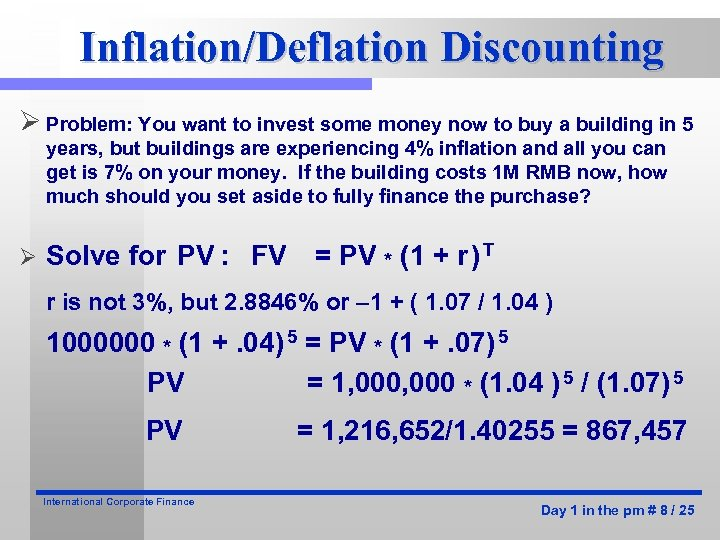Inflation/Deflation Discounting Ø Problem: You want to invest some money now to buy a building in 5 years, but buildings are experiencing 4% inflation and all you can get is 7% on your money. If the building costs 1 M RMB now, how much should you set aside to fully finance the purchase? Ø Solve for PV : FV = PV * (1 + r ) T r is not 3%, but 2. 8846% or – 1 + ( 1. 07 / 1. 04 ) 1000000 * (1 +. 04) 5 = PV * (1 +. 07) 5 PV = 1, 000 * (1. 04 ) 5 / (1. 07) 5 PV International Corporate Finance = 1, 216, 652/1. 40255 = 867, 457 Day 1 in the pm # 8 / 25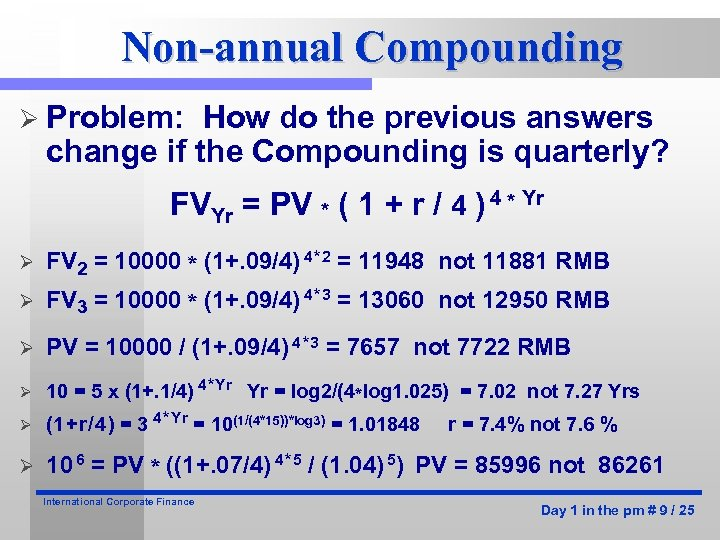Non-annual Compounding Ø Problem: How do the previous answers change if the Compounding is quarterly? FVYr = PV * ( 1 + r / 4 ) 4 * Yr Ø FV 2 = 10000 * (1+. 09/4) 4 * 2 = 11948 not 11881 RMB Ø FV 3 = 10000 * (1+. 09/4) 4 * 3 = 13060 not 12950 RMB Ø PV = 10000 / (1+. 09/4) 4 * 3 = 7657 not 7722 RMB Ø 10 = 5 x (1+. 1/4) 4 * Yr Yr = log 2/(4*log 1. 025) = 7. 02 not 7. 27 Yrs Ø (1 + r / 4 ) = 3 4 * Yr = 10(1/(4*15))*log 3) = 1. 01848 Ø 10 6 = PV * ((1+. 07/4) 4 * 5 / (1. 04) 5) PV = 85996 not 86261 International Corporate Finance r = 7. 4% not 7. 6 % Day 1 in the pm # 9 / 25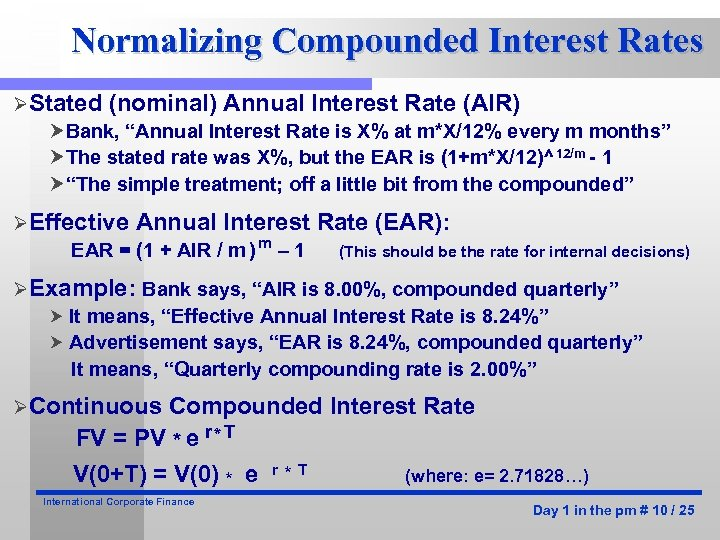Normalizing Compounded Interest Rates ØStated (nominal) Annual Interest Rate (AIR) Bank, “Annual Interest Rate is X% at m*X/12% every m months” The stated rate was X%, but the EAR is (1+m*X/12)^12/m - 1 “The simple treatment; off a little bit from the compounded” ØEffective Annual Interest EAR = (1 + AIR / m ) m – 1 Rate (EAR): (This should be the rate for internal decisions) ØExample: Bank says, “AIR is 8. 00%, compounded quarterly” It means, “Effective Annual Interest Rate is 8. 24%” Advertisement says, “EAR is 8. 24%, compounded quarterly” It means, “Quarterly compounding rate is 2. 00%” ØContinuous Compounded Interest Rate FV = PV * e r * T V(0+T) = V(0) * e International Corporate Finance r * T (where: e= 2. 71828…) Day 1 in the pm # 10 / 25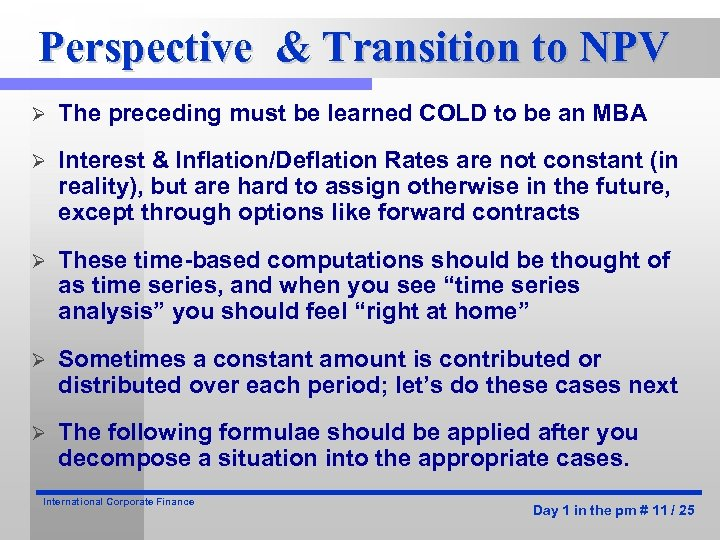Perspective & Transition to NPV Ø The preceding must be learned COLD to be an MBA Ø Interest & Inflation/Deflation Rates are not constant (in reality), but are hard to assign otherwise in the future, except through options like forward contracts Ø These time-based computations should be thought of as time series, and when you see “time series analysis” you should feel “right at home” Ø Sometimes a constant amount is contributed or distributed over each period; let’s do these cases next Ø The following formulae should be applied after you decompose a situation into the appropriate cases. International Corporate Finance Day 1 in the pm # 11 / 25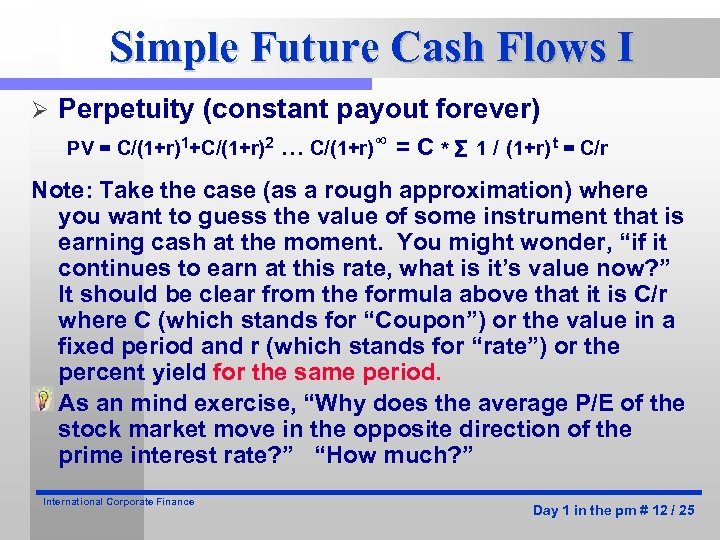Simple Future Cash Flows I Ø Perpetuity (constant payout forever) PV = C/(1+r)1+C/(1+r)2 … C/(1+r)∞ = C * Σ 1 / (1+r) t = C/r Note: Take the case (as a rough approximation) where you want to guess the value of some instrument that is earning cash at the moment. You might wonder, “if it continues to earn at this rate, what is it’s value now? ” It should be clear from the formula above that it is C/r where C (which stands for “Coupon”) or the value in a fixed period and r (which stands for “rate”) or the percent yield for the same period. As an mind exercise, “Why does the average P/E of the stock market move in the opposite direction of the prime interest rate? ” “How much? ” International Corporate Finance Day 1 in the pm # 12 / 25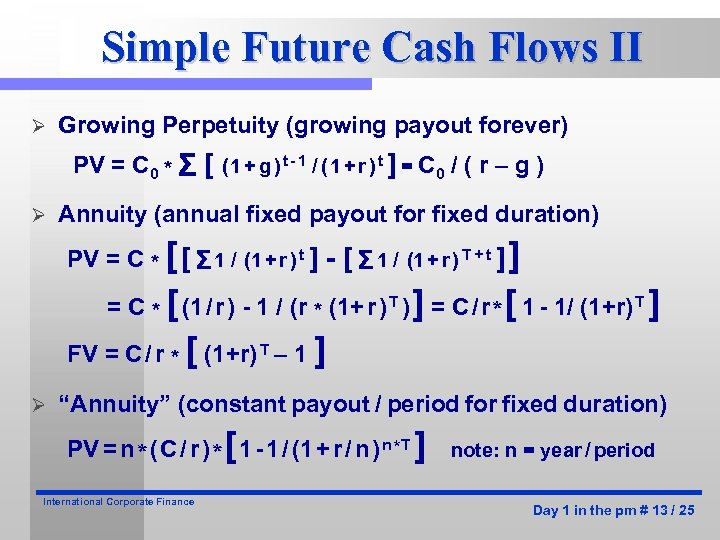Simple Future Cash Flows II Ø Growing Perpetuity (growing payout forever) PV = C 0 * Σ [ ( 1 + g ) t - 1 / ( 1 + r ) t ] = C 0 / ( r – g ) Ø Annuity (annual fixed payout for fixed duration) PV = C * [ [ Σ 1 / (1 + r ) t ] - [ Σ 1 / (1 + r ) T + t ] ] = C * [ (1 / r ) - 1 / (r * (1+ r ) T ) ] = C / r * [ 1 - 1/ (1+r) T ] FV = C / r * [ (1+r) T – 1 ] Ø “Annuity” (constant payout / period for fixed duration) PV = n * ( C / r ) * [ 1 - 1 / (1 + r / n ) n * T ] International Corporate Finance note: n = year / period Day 1 in the pm # 13 / 25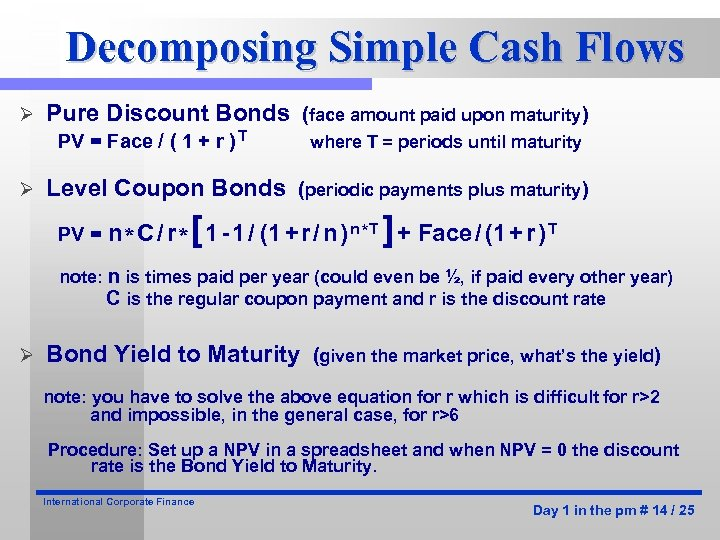Decomposing Simple Cash Flows Ø Pure Discount Bonds (face amount paid upon maturity) PV = Face / ( 1 + r ) T Ø where T = periods until maturity Level Coupon Bonds (periodic payments plus maturity) PV = n * C / r * [ 1 - 1 / (1 + r / n ) n * T ] + Face / (1 + r ) T note: n is times paid per year (could even be ½, if paid every other year) C is the regular coupon payment and r is the discount rate Ø Bond Yield to Maturity (given the market price, what’s the yield) note: you have to solve the above equation for r which is difficult for r>2 and impossible, in the general case, for r>6 Procedure: Set up a NPV in a spreadsheet and when NPV = 0 the discount rate is the Bond Yield to Maturity. International Corporate Finance Day 1 in the pm # 14 / 25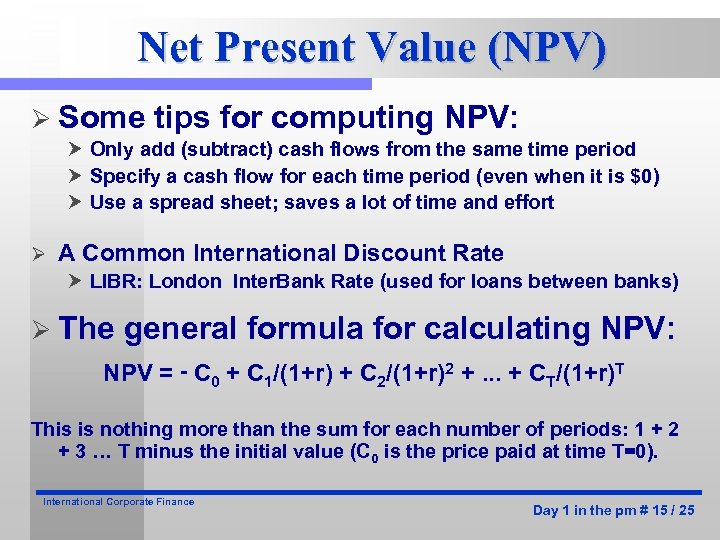Net Present Value (NPV) Ø Some tips for computing NPV: Only add (subtract) cash flows from the same time period Specify a cash flow for each time period (even when it is \$0) Use a spread sheet; saves a lot of time and effort Ø A Common International Discount Rate LIBR: London Inter. Bank Rate (used for loans between banks) Ø The general formula for calculating NPV: NPV = - C 0 + C 1/(1+r) + C 2/(1+r)2 +. . . + CT/(1+r)T This is nothing more than the sum for each number of periods: 1 + 2 + 3 … T minus the initial value (C 0 is the price paid at time T=0). International Corporate Finance Day 1 in the pm # 15 / 25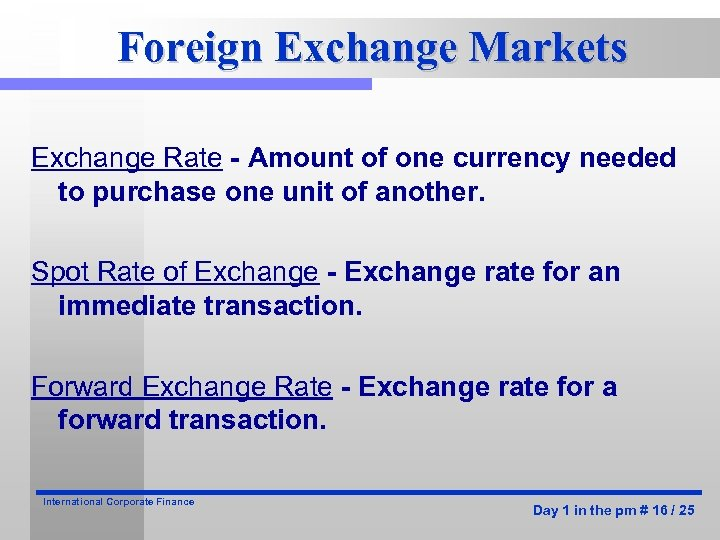Foreign Exchange Markets Exchange Rate - Amount of one currency needed to purchase one unit of another. Spot Rate of Exchange - Exchange rate for an immediate transaction. Forward Exchange Rate - Exchange rate for a forward transaction. International Corporate Finance Day 1 in the pm # 16 / 25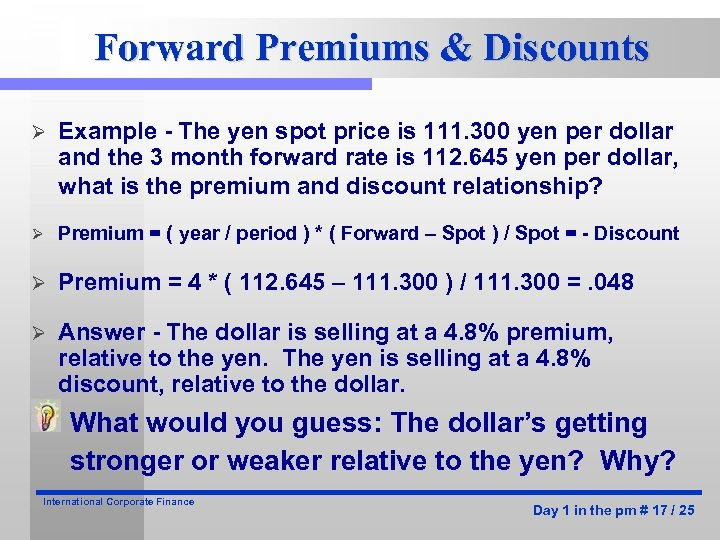Forward Premiums & Discounts Ø Example - The yen spot price is 111. 300 yen per dollar and the 3 month forward rate is 112. 645 yen per dollar, what is the premium and discount relationship? Ø Premium = ( year / period ) * ( Forward – Spot ) / Spot = - Discount Ø Premium = 4 * ( 112. 645 – 111. 300 ) / 111. 300 =. 048 Ø Answer - The dollar is selling at a 4. 8% premium, relative to the yen. The yen is selling at a 4. 8% discount, relative to the dollar. What would you guess: The dollar’s getting stronger or weaker relative to the yen? Why? International Corporate Finance Day 1 in the pm # 17 / 25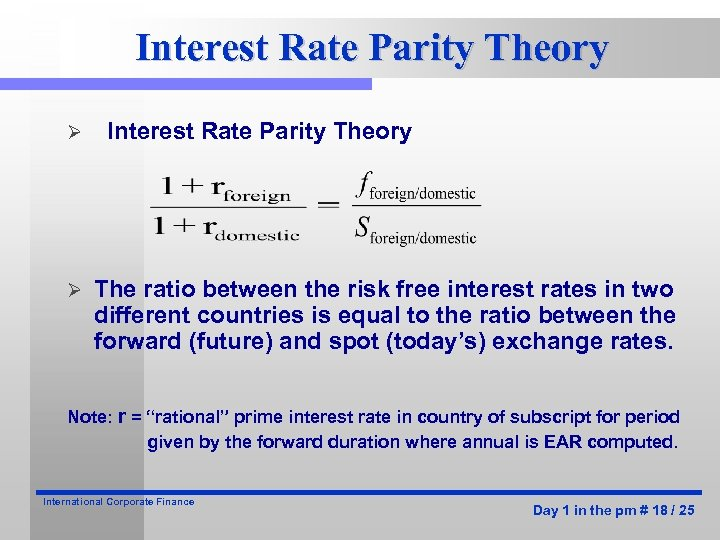Interest Rate Parity Theory Ø Ø Interest Rate Parity Theory The ratio between the risk free interest rates in two different countries is equal to the ratio between the forward (future) and spot (today’s) exchange rates. Note: r = “rational” prime interest rate in country of subscript for period given by the forward duration where annual is EAR computed. International Corporate Finance Day 1 in the pm # 18 / 25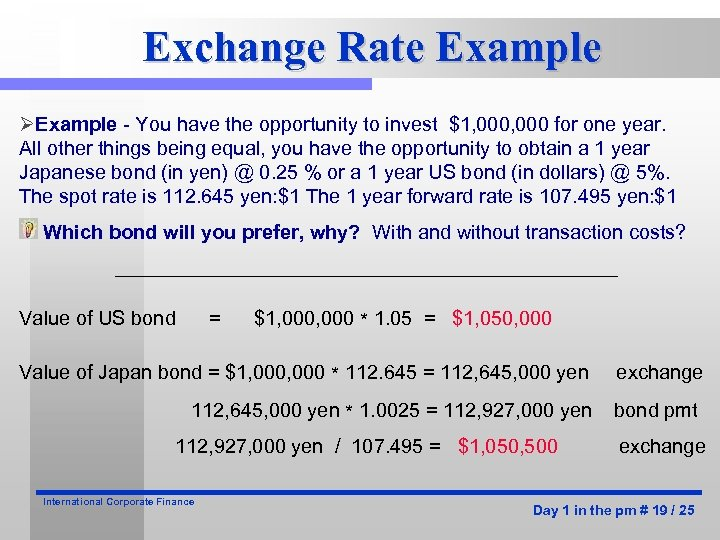Exchange Rate Example ØExample - You have the opportunity to invest \$1, 000 for one year. All other things being equal, you have the opportunity to obtain a 1 year Japanese bond (in yen) @ 0. 25 % or a 1 year US bond (in dollars) @ 5%. The spot rate is 112. 645 yen: \$1 The 1 year forward rate is 107. 495 yen: \$1 Which bond will you prefer, why? With and without transaction costs? Value of US bond = \$1, 000 * 1. 05 = \$1, 050, 000 Value of Japan bond = \$1, 000 * 112. 645 = 112, 645, 000 yen exchange 112, 645, 000 yen * 1. 0025 = 112, 927, 000 yen bond pmt 112, 927, 000 yen / 107. 495 = \$1, 050, 500 International Corporate Finance exchange Day 1 in the pm # 19 / 25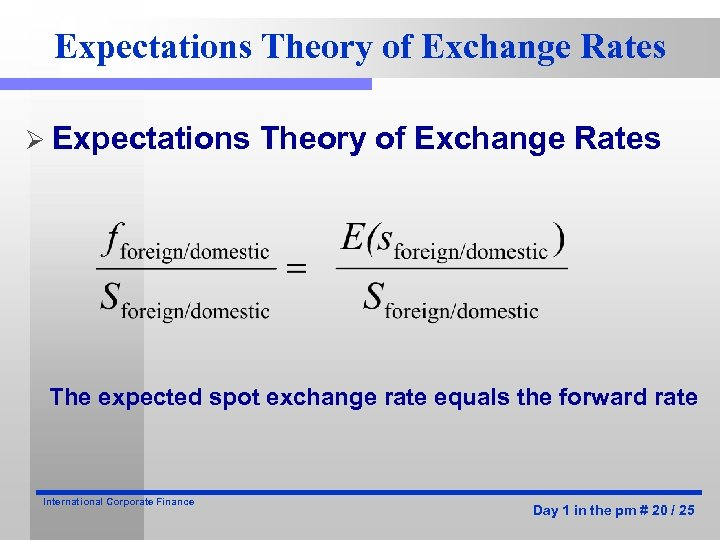Expectations Theory of Exchange Rates Ø Expectations Theory of Exchange Rates The expected spot exchange rate equals the forward rate International Corporate Finance Day 1 in the pm # 20 / 25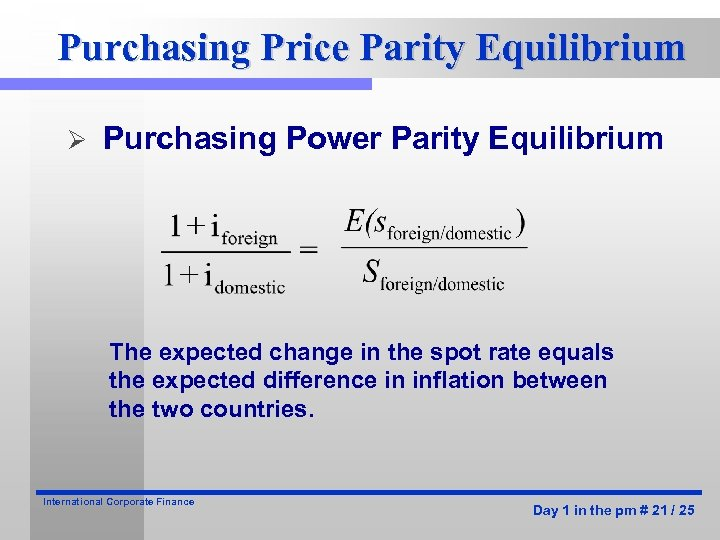Purchasing Price Parity Equilibrium Ø Purchasing Power Parity Equilibrium The expected change in the spot rate equals the expected difference in inflation between the two countries. International Corporate Finance Day 1 in the pm # 21 / 25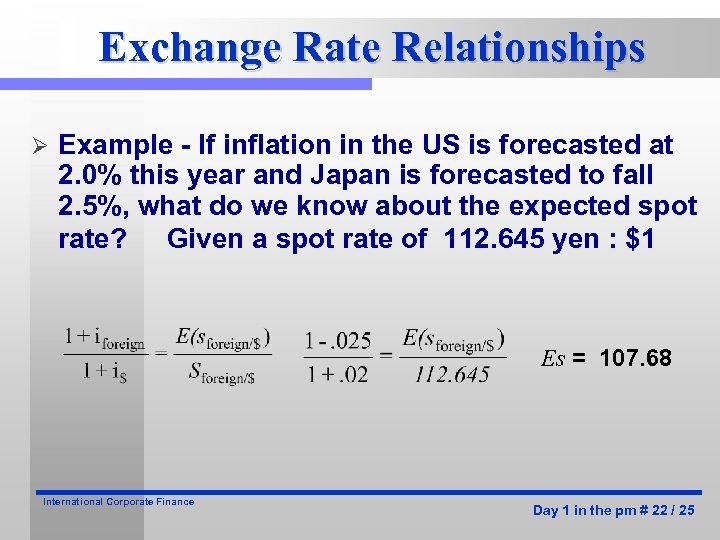Exchange Rate Relationships Ø Example - If inflation in the US is forecasted at 2. 0% this year and Japan is forecasted to fall 2. 5%, what do we know about the expected spot rate? Given a spot rate of 112. 645 yen : \$1 Es = 107. 68 International Corporate Finance Day 1 in the pm # 22 / 25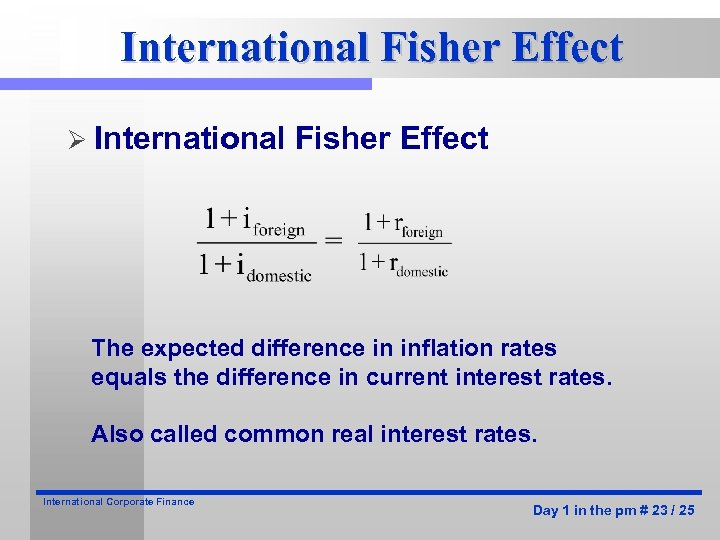International Fisher Effect Ø International Fisher Effect The expected difference in inflation rates equals the difference in current interest rates. Also called common real interest rates. International Corporate Finance Day 1 in the pm # 23 / 25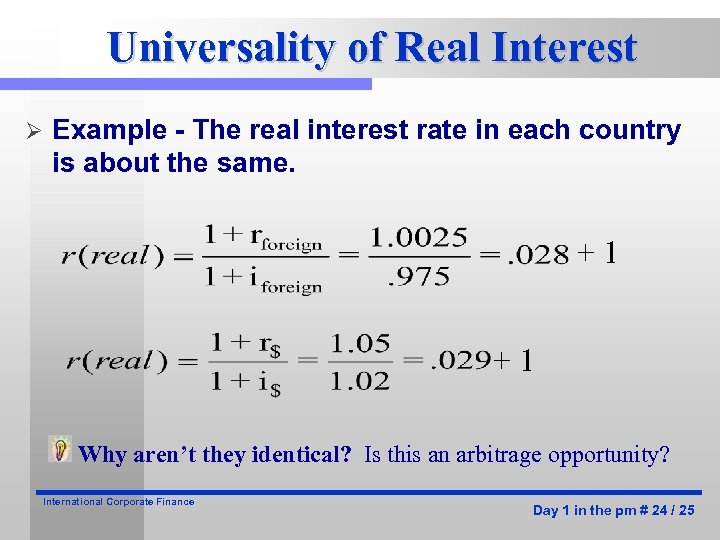Universality of Real Interest Ø Example - The real interest rate in each country is about the same. +1 +1 Why aren’t they identical? Is this an arbitrage opportunity? International Corporate Finance Day 1 in the pm # 24 / 25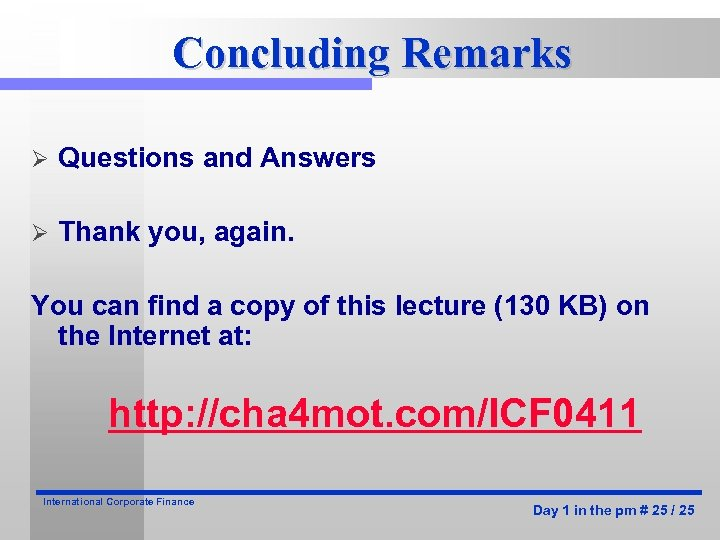Concluding Remarks Ø Questions and Answers Ø Thank you, again. You can find a copy of this lecture (130 KB) on the Internet at: http: //cha 4 mot. com/ICF 0411 International Corporate Finance Day 1 in the pm # 25 / 25